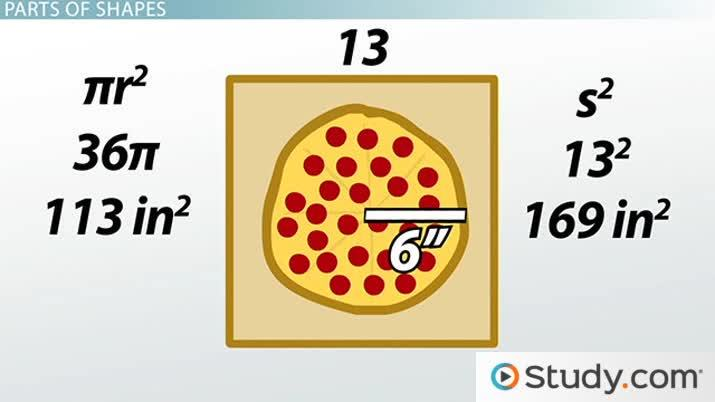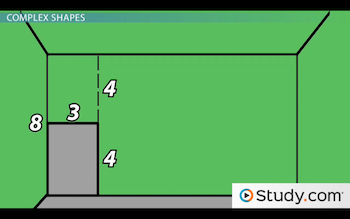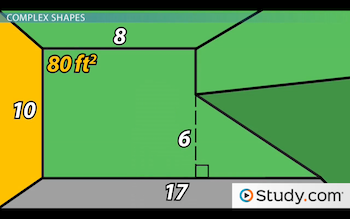# Area of Complex Figures

Lesson Transcript
Instructor: Jeff Calareso

Jeff teaches high school English, math and other subjects. He has a master's degree in writing and literature.

Finding the area of shapes isn't always as simple as it is with squares and rectangles. In this lesson, you'll learn how to find the area of complex figures. This includes finding the area of unusual shapes and finding the area of parts of shapes. Updated: 10/31/2019

## Area in the Real World

Let's say you need to paint some rooms in your house. How much paint will you need? You need to know the surface area of the walls. If you live in a really boring house, all your walls are neat rectangles and squares. But, maybe there are angled ceilings. Or windows - you don't need paint to cover the windows, do you?

In fact, determining the area of shapes in the real world can seem more complicated than your standard 'area of a rectangle' geometry problem. So, let's look at how you can solve area questions with some unusual shapes. It might just save you an extra trip to the store for more paint.An error occurred trying to load this video.

Try refreshing the page, or contact customer support.

Coming up next: Circles: Area and Circumference

### You're on a roll. Keep up the good work!

Replay
Your next lesson will play in 10 seconds
• 0:06 Area in the Real World
• 0:39 Review of Basic Shapes
• 2:10 Complex Shapes
• 3:56 Parts of Shapes
• 6:03 Lesson Summary
Save Save

Want to watch this again later?

Timeline
Autoplay
Autoplay
Speed Speed

## Review of Basic Shapes

Before we get into complex figures, let's quickly review the area formulas we'll need. First up is the rectangle. A rectangle is a four-sided shape where all the angles are 90 degrees. With rectangles, the sides opposite of each other are equal. Remember that - it's important. The area of a rectangle is width times height. In other words, multiply the long side by the short side, and you're done!

Next is the square. A square is a type of rectangle where all the sides are equal in length. Therefore, you only need to know one side's length to know all of the sides. That's going to come in handy. Also, the area of a square is s^2, where s is the length of one of the sides. So again, if you know one side of a square, you have all the power.

Next is the triangle. The area of a triangle is 1/2 * b * h, or 1/2 * base * height. So, you need to know more about triangles to find their areas. But, there are a ton of useful triangle facts that are helpful. For example, in a triangle with one right angle, you can use the Pythagorean theorem, a^2 + b^2 = c^2, to determine the length of a missing side.

Finally, there are circles. If you've ever stared at an extra-large pizza and wondered how much space it takes up, then you've thought about the area of a circle. The formula is pi * r^2, where r is the radius of a circle. That's 3.14 pi, by the way, not pizza pie. Okay, with those formulas in mind, let's get to the task at hand.

## Complex Shapes

Let's look at a wall you need to paint.Okay, you know the measurements, but how do you account for that space in the corner? Well, what if you draw a line above the space in the corner? A complex shape just became two rectangles, and you're a rectangle ninja. But wait, do you know the measurements for these new shapes? You do!

The small rectangle has a width of 3 feet. And, if the height above it is 4 feet, and the whole wall is 8 feet, then the height of the small rectangle is 4 feet. That rectangle must be 3 times 4, or 12 square feet. As for the big rectangle, again, its height is 8 feet. The width is 10 feet of the whole wall minus the 3 feet of the small rectangle, so it's 7 feet. And, 8 times 7 is 56 square feet. Add 56 and 12, and you get 68 square feet.

Let's look at another. Here's one from that room with a tiny corner:You avoid it because sometimes there are spiders in that corner, but you don't want to have to tell people you didn't paint the whole wall because of spiders. So, what can we do? If we draw a line perpendicular to the floor, we have a rectangle and a right triangle. Awesome. That rectangle is just 8 by 10 feet, or 80 square feet.

And that triangle? You know it's 6 feet high, because your 61` friend always hits his head when he offers to kill the spiders for you. But, how long is it? Well, the whole wall is 17 feet. And, the rectangle is 8 feet wide. So, it's 17 minus 8, or 9 feet. What's the area of a triangle? 1/2 * b * h. So, it's 1/2 * 9 * 6, or 27 square feet. This whole wall, spider territory and all, is 80 plus 27, or 107 square feet.

To unlock this lesson you must be a Study.com Member.

### Register to view this lesson

Are you a student or a teacher?